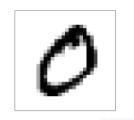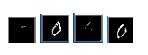# keras 特征图可视化实例(中间层)

更新时间：2020年01月24日 09:48:33   作者：wanbeila

```def simple_cnn():
input_data = Input(shape=(28, 28, 1))
x = Conv2D(64, kernel_size=3, padding='same', activation='relu', name='conv1')(input_data)
x = MaxPooling2D(pool_size=2, strides=2, name='maxpool1')(x)
x = Conv2D(32, kernel_size=3, padding='same', activation='relu', name='conv2')(x)
x = MaxPooling2D(pool_size=2, strides=2, name='maxpool2')(x)
x = Dropout(0.25)(x)
# 获得最后一层卷积层的输出
# 添加自己的全连接
x = Flatten(name='flatten')(x)
x = Dense(128, activation='relu', name='fc1')(x)
x = Dropout(0.25)(x)
x = Dense(10, activation='softmax', name='fc2')(x)
model = Model(inputs=input_data, outputs=x)``````input_data = Input(shape=(28, 28, 1))
x = Conv2D(64, kernel_size=3, padding='same', activation='relu', name='conv1')(input_data)
x = MaxPooling2D(pool_size=2, strides=2, name='maxpool1')(x)
x = Conv2D(32, kernel_size=3, padding='same', activation='relu', name='conv2')(x)
x = MaxPooling2D(pool_size=2, strides=2, name='maxpool2')(x)
x = Dropout(0.25)(x)
x = Flatten(name='flatten')(x)
x = Dense(128, activation='relu', name='fc1')(x)
x = Dropout(0.25)(x)
x = Dense(10, activation='softmax', name='fc2')(x)
model = Model(inputs=input_data, outputs=x)

test_img = load_img('test.png', color_mode='grayscale', target_size=(28, 28))
test_img = np.array(test_img)
test_img = np.expand_dims(test_img, axis=0)
test_img = np.expand_dims(test_img, axis=3)

conv1_layer = Model(inputs=input_data, outputs=model.get_layer(index=1).output)

conv1_output = conv1_layer.predict(test_img)

for i in range(64):
show_img = conv1_output[:, :, :, i]
print(show_img.shape)
show_img.shape = [28,28]
cv2.imshow('img', show_img)
cv2.waitKey(0)
```